Sample Size Computation

The SEQDESIGN procedure assumes that the data are from a multivariate normal distribution and the sequence of the standardized test statistics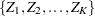has the following canonical joint distribution:

•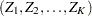is multivariate normal

•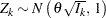•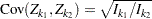,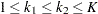where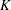is the total number of stages and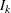is the information available at stage.

If the test statistic is computed from the data that are not from a normal distribution, such as a binomial distribution, then it is assumed that the test statistic is computed from a large sample such that the statistic has an approximately normal distribution.

In a typical clinical trial, the sample size required depends on the Type I error probability level, alternative reference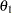, power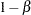, and variance of the response variable. Given a one-sided null hypothesis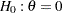with an upper alternative hypothesis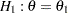, the information required for a fixed-sample test is given by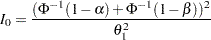The parameter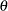and the subsequent alternative referencedepend on the test specified in the clinical trial. For example, suppose you are comparing two binomial populations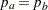; then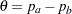is the difference between two proportions if the proportion difference statistic is used, and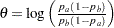, the log odds ratio for the two proportions if the log odds ratio statistic is used.

If the maximum likelihood estimate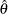from the likelihood function can be derived, then the asymptotic variance foris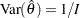, whereis Fisher information for. The resulting statisticcorresponds to the MLE statistic scale as specified in the BOUNDARYSCALE=MLE option in the PROC SEQDESIGN statement,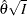corresponds to the standardized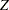scale (BOUNDARYSCALE=STDZ), and
corresponds to the score statistic scale (BOUNDARYSCALE=SCORE).

Alternatively, if the score statistic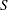is derived in a statistical procedure, it can be used as the test statistic and its asymptotic variance is given by Fisher information,. In this case,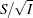corresponds to the standardizedscale and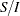corresponds to the MLE statistic scale.

For a group sequential trial, the maximum information
is derived in the SEQDESIGN procedure with the specified,, and. With the maximum information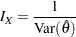the sample size required for a specified test statistic in the trial can be evaluated or estimated from the known or estimated variance of the response variable. Note that different designs might produce different maximum information levels for the same hypothesis, and this in turn might require a different number of observations for the trial.

If each observation in the data set provides one unit of information in a hypothesis testing, such as a one-sample test for the mean, the required sample size for the sequential design can be derived from the maximum information. However, for a survival analysis, an individual in the survival time data might provide only partial information because of censoring. In this case, the required number of events can be derived from the maximum information. With addition accrual information, the sample size can also be computed.

The SEQDESIGN procedure provides sample size computation for some one-sample and two-sample tests in the SAMPLESIZE statement. It also provides sample size computation for tests of a parameter in regression models such as normal regression, logistic regression, and proportional hazards regression. In addition, the procedure can also compute the required sample size or number of events from the corresponding number in the fixed-sample design.

Table 80.11 lists the options available in the SAMPLESIZE statement.

Table 80.11 SAMPLESIZE Statement Options

Option

Description

Fixed-Sample Models

INPUTNOBS

specifies sample size for fixed-sample design

INPUTNEVENTS

specifies number of events for fixed-sample design

One-Sample Models

ONESAMPLEMEAN

specifies one-sampletest for mean

ONESAMPLEFREQ

specifies one-sample test for binomial proportion

Two-Sample Models

TWOSAMPLEMEAN

specifies two-sampletest for mean difference

TWOSAMPLEFREQ

specifies two-sample test for binomial proportions

TWOSAMPLESURVIVAL

specifies log-rank test for two survival distributions

Regression Models

REG

specifies test for a regression parameter

LOGISTIC

specifies test for a logistic regression parameter

PHREG

specifies test for a proportional hazards regression parameter

The MODEL=INPUTNOBS and MODEL=INPUTNEVENTS options are described next, and the remaining options are described in the next three sections.

### Input Sample Size for Fixed-Sample Design

The MODEL=INPUTNOBS option derives the sample size required for a group sequential trial from the sample size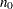for the corresponding fixed-sample design. With the N=option specifying the sample sizefor a fixed-sample design, the sample size required for a group sequential trial is then computed as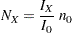whereis the maximum information for the group sequential design and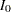is the information for the corresponding fixed-sample design. The information ratio betweenandis derived in the SEQDESIGN procedure.

The SAMPLE=ONE option specifies a one-sample test, and the SAMPLE=TWO option specifies a two-sample test. For a two-sample test, the WEIGHT= option specifies the sample size allocation weights for the two groups.

### Input Number of Events for Fixed-Sample Design

The MODEL=INPUTNOBS option derives the number of events required for a group sequential trial from the number of events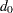for the corresponding fixed-sample design. With the D=option specifies the number of eventsfor a fixed-sample survival analysis, the number of events required for a group sequential trial is then computed as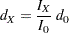whereis the maximum information for the group sequential design andis the information for the corresponding fixed-sample design. The information ratio betweenandis derived in the SEQDESIGN procedure.

The SAMPLE=ONE option specifies a one-sample test, and the SAMPLE=TWO option specifies a two-sample test. For a two-sample test, the WEIGHT= option specifies the sample size allocation weights for the two groups.

With the computed number of events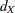for a group sequential survival design, the required total sample size and sample size at each stage can be derived with specifications of hazard rates, accrual rate, and accrual time.

For a study group, if the hazard rateis constant, corresponding to an exponential survival distribution, and the individual accrual is uniform in the accrual time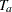with a constant accrual rate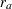, Kim and Tsiatis (1990, pp. 83–84) show that the expected number of events by timeis given by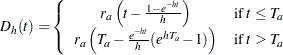For a one-sample design, such as a proportional hazards regression, the expected number of events by timeis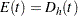, whereis the hazard rate for the group. For a two-sample design, such as a log-rank test for two survival distributions, the expected number of events by timeis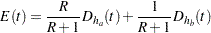where
and
are hazard rates in groups A and B, respectively, and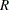is the ratio of the sample size allocation weights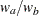.

If the accrual rateis specified without the accrual time, follow-up time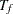, and total study time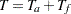, the SEQDESIGN procedure computes the minimum and maximum accrual times from the following equation, as described in Kim and Tsiatis (1990, p. 85):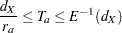If the accrual rateis specified with one of the three time parameters—the accrual time, follow-up time, and total study time—then the other two time parameters are computed in the SEQDESIGN procedure. Similarly, if the accrual rateis not specified, but two of the three time parameters are specified, then the accrual rate is derived in the SEQDESIGN procedure.

With the accrual rateand the accrual time, the total sample size is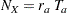At each stage, the number of events is given by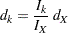The corresponding time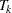can be derived from the equation for the expected number of events,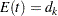, and the resulting sample size is computed as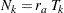The following three sections describe examples of test statistics with their resulting information levels, which can then be used to derive the required sample size. The maximum likelihood estimators are used for all tests except to compare two survival distributions with a log-rank test, where a score statistic is used.